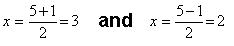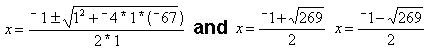Sheri uses the quadratic formula to find the missing base for Don's age

Don had Sheri solve x2 - 5x + 6 = 0  using the quadratic formula. She knew what the two answers were {2,3}, but Don helped her to substitute in the formula, then get the two answers:Don had Sheri list what a, b and c are in x2 - 5x + 6 = 0;  a = 1, b=

- 5 and c=6. a=1 was not obvious; they talked about what times x2 = x2 ? 1. Don substituted these values of a, b, and c into the formula:They gotThe ' means "plus or minus" and this gives the two answers as shown above. Sheri then solved another quadratic equation (for which she knew the answers), using the using the formula, then solved x2 + x + 4 = 71 or x2 + x - 67 = 0 to find the base for Don's age:These two exact answers are approximately 7.700609733... and -8.700609733.  Sheri got the first answer, but didn't have time to get the second one. Notice that in using the calculator to do find the base earlier, Sheri got 7.7, which was very close to the answer using the quadratic equation. So Don's "age" of 114 is in base 7.7 to get his real age of 71!

There are many good questions that Don thinks of now..what do you think?

Sheri writes 64 using exponents
Sheri solves the quadratic equation x2 - x - 1 = 0
Sheri finds the base for Don's age of   114? = 7110
Sheri finds the measure of an inscribed angle
Sheri uses binary numerals to make the Magic Number Game cards
Sheri changes the shape of a dog using matrices
Sheri reduces the size of a shell using the pantograph
Sheri moves a parabola and finds the equation
Sheri finds the ratio of The Volume of a Pyramid / The Volume of a Cube (3 ways)
Sheri works with the sand pendulum
Sheri figures out a rule for The Tower Puzzle
Sheri starts Trig

To other discoveries

To order Don's materials
Mathman home